• Call Now

1800-102-2727•

# Mirrors, types, uses, mirror formula, practice problems, FAQs

You might have heard that every person has a look alike person somewhere in the world. Did you meet with your lookalike? No problem, you can see him or her in your home. Just take a mirror and put it in front of you and you will see a person who looks like you. This is your mirror image. It can be bigger, smaller or the same size depending upon the choice of mirror. Let's know more about mirrors !

Table of content

## What is a mirror?

A mirror is an optical device that reflects the light incident on it. It is used to see the image of objects. There are different kinds of mirrors, all have their own function. Basically the mirror is the transparent glass which has two surfaces, one of these surfaces is covered with a reflecting material and the other is used for reflection .

## Terms related to mirror

1. Incident ray : The ray comes from an object and hits the mirror.
2. Reflected rays : The ray that reflects after hitting the reflecting surface.
3. Normal : It is the line perpendicular to the reflecting surface.
4. Angle of incidence : The angle between incident ray and normal to the surface at the point of incidence is known as angle of incidence.
5. Angle of reflection : The angle between reflected ray and normal to the surface at the point of incidence is considered as angle of reflection.
6. Angle of deviation : The angle between the direction of incident ray and direction of reflected ray is known as angle of deviation. Angle of deviation is given by $\delta =\pi -2i$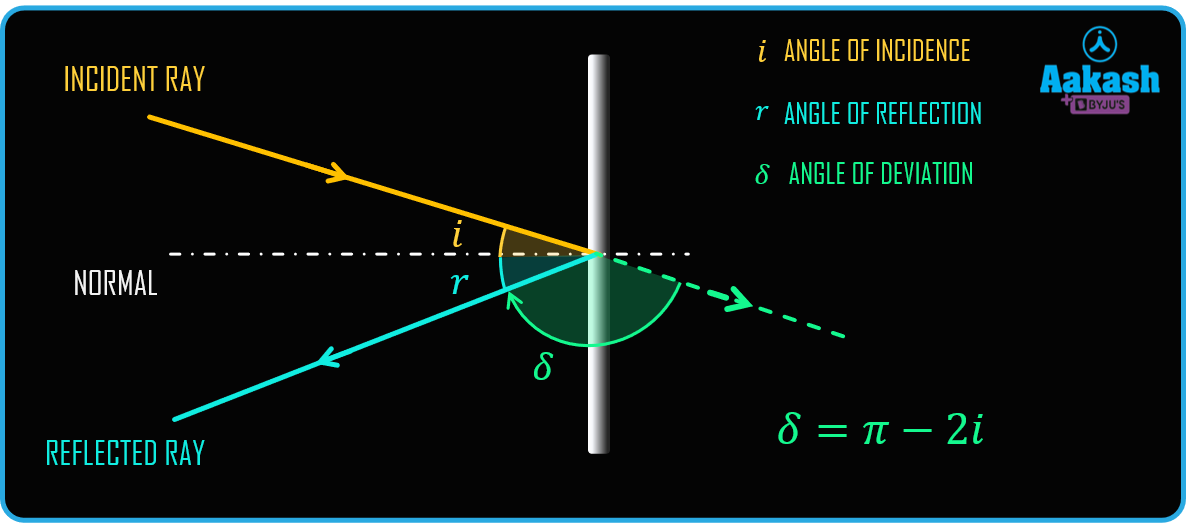## Laws of reflection

The light ray reflected from a mirror obeys the following two laws of reflection:

1. The incident angle is equal to the angle of reflection (i = r).
2. The incident ray, the reflected ray, and the normal to the reflecting surface at the point of incidence are coplanar.

## Types of mirror

The mirrors can be broadly classified as plane mirror and spherical mirror. Spherical mirror is further classified into concave mirror and convex mirror.

### Plane mirror

A plane mirror is a polished glass plate. One surface of the plane mirror is coated with a reflecting material and the other surface is used to reflect the light. It follows the laws of reflection. The plane mirror is shown in figure.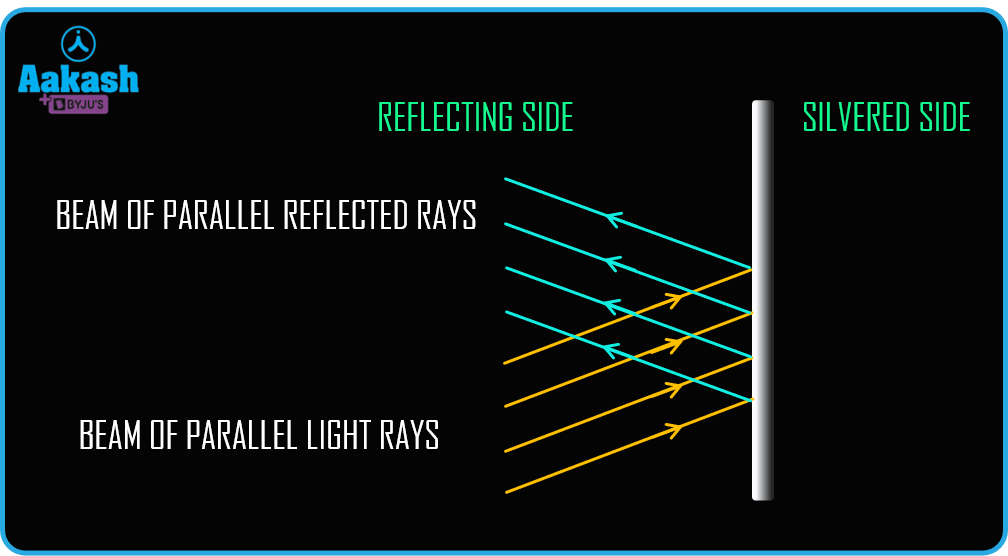Image formed by plane mirror

Consider a tree situated in front of a plane mirror. To form the image of this tree in the plane mirror first consider a point A. A light ray which falls on the plane mirror perpendicularly will return back on the same path. Now a ray of light incident at an angle on the plane mirror will reflect at the same angle of reflection. If we extend both the reflecting rays they will intersect at a point A’ . This will be the image of point A. Similarly all the points on the tree will form an image behind the mirror. If we combine all these images we will get the complete image of the tree. The image will be virtual, erect and equal in size of the object and will be the same distance as the object from the plane mirror.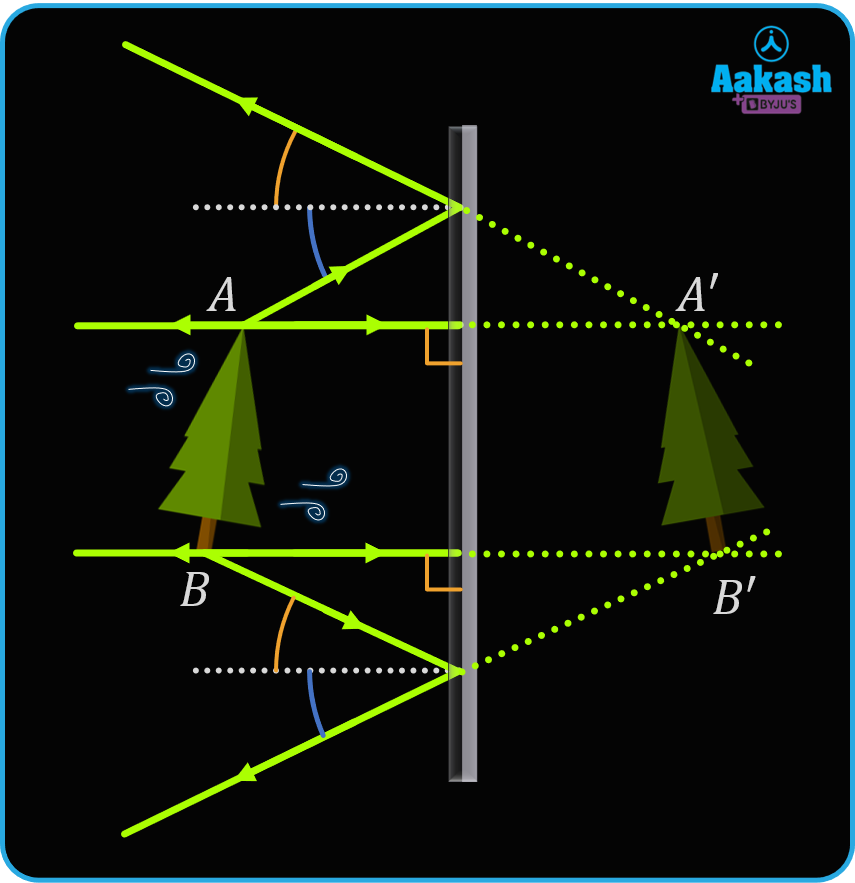### Spherical mirror

A spherical mirror is a portion of hollow sphere whose one surface is polished with the reflecting material. Spherical mirrors can be of two types- Concave mirror and convex mirror.

1. Concave mirror - A spherical mirror whose outer surface is silvered and inner surface is used for reflection is called concave mirror. In concave mirror, the reflecting surface is located towards the center of the sphere. It can produce both real and virtual images.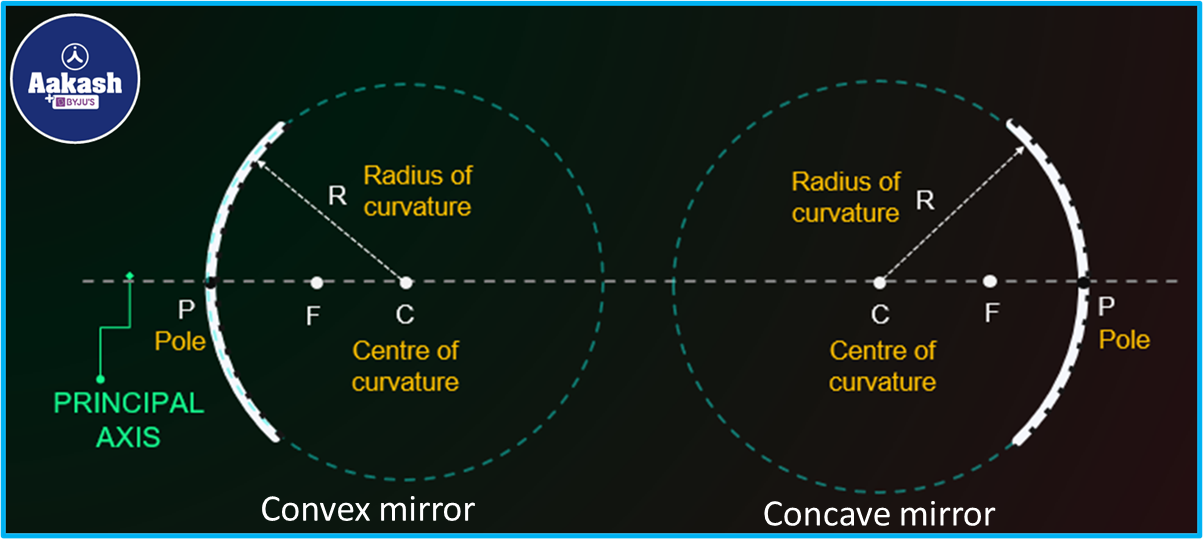1. Convex mirror- A spherical mirror whose inner surface is silvered and outer surface is used for reflection is called convex mirror. In a convex mirror the reflecting surface is away from the center of the sphere. It produces virtual images for real objects.

## Mirror formula

Mirror formula is an equation relating object distance and image distance with focal length. Mathematically it is given as

$\frac{1}{f}=\frac{1}{u}+\frac{1}{v}$

Where, u = Distance of the object from the pole
v = Distance of the image from the pole
f = Focal length of the mirror

Sign convention : The direction of the incident light ray is taken as positive and the direction opposite to the incident ray is taken as negative.

## Uses of mirror

• Plane mirror - Plane mirror produces virtual, upright and equal size of image. It can be used for
1. Dressing purpose
2. dental
3. solar cooker
• Concave mirror - As concave mirror converge the light ray and forms different images for different positions, so we can use it as
1. Shaving mirror : when an object is placed inside the focal distance a virtual and large image is produced.
2. Head light of the car : if we put a light source on the focus, a parallel beam of light will produce.
• Convex mirror - Convex mirror always produce virtual, small and erect image, so it can be used for following purpose
1. Car’s rear view mirror : convex mirror covers the large area for rear view.
2. At the junctions of the roads.

## Practice problems

Q. A light ray is incident on a plane mirror at an angle 24o as shown, find the angle of reflection.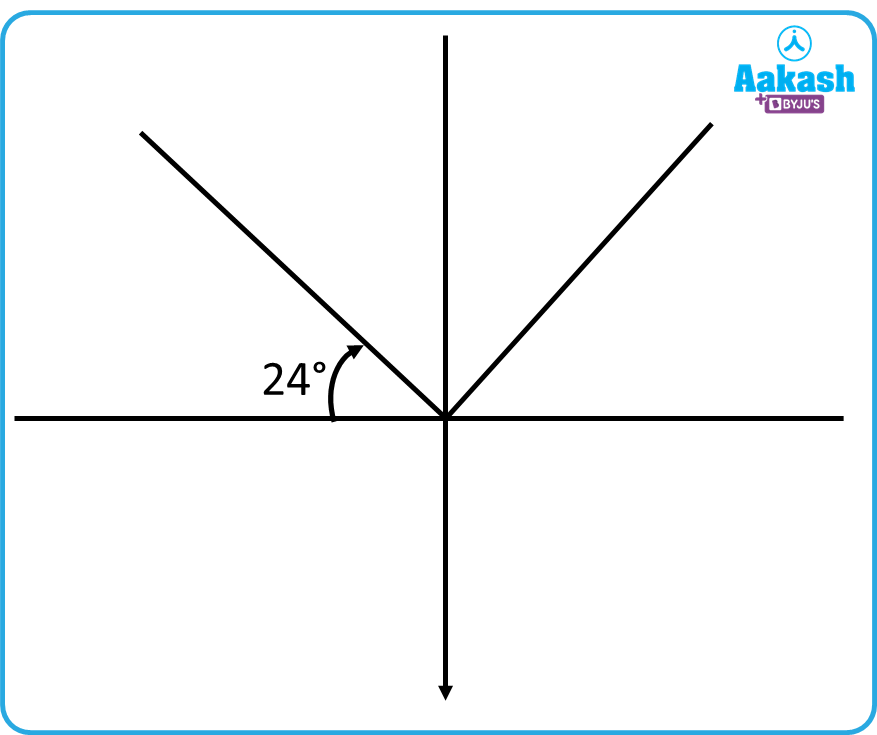A. The Incident angle i = 90- 24= 66o
According to laws of reflection i = r
So the angle of reflection r = 66o

Q. An object is placed 30 cm in front of a mirror and produces a real image at 20 cm. Find the focal length of the mirror and the nature of the mirror.

A. Given distance of object u = -30 cm
Distance of image v = -20 cm
Using the mirror formula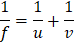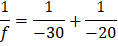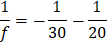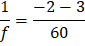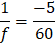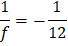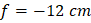Focal length of mirror is 12 cm. The focal length is negative, so it is a concave mirror.

Q. A ray of light incident at an angle of incidence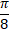on a plane mirror. Find the angle of deviation of the ray.

A. Given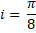We know the relation for angle of deviation is given as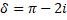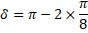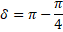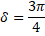Hence angle of deviation is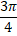.

Q. A concave mirror is producing a real image at a distance of 23 cm in front of the mirror. If the focal distance of the mirror is 20 cm, what is the location of the object?

A. v=-23 cm and f=-20 cm

Now using the mirror formula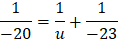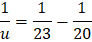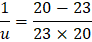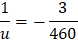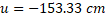Hence the object will be placed at 153.33 cm in front of the mirror.

## FAQs

Q. What is the working of a mirror?
A. When a ray of light comes into contact with the reflecting surface of a mirror, at microscopic level the molecule of polished material gets excited by absorbing photons which makes the atom unstable. For getting stable, the molecule reflects those photons.

Q. Write a difference between incident ray and reflecting ray.
A. A light ray which comes from the source and reaches to the reflecting surface is known as incident ray. On the other hand rays formed after the reflection and goes from mirror to image are known as reflecting rays.

Q. What is an object for a mirror?
A. Object is the point where the incident rays meet.

Q. What is an image for a mirror?
A. Image is the point where the reflected rays meet.

Q. What is the fundamental property of the mirror?
A. Reflection of light is the fundamental property of a mirror.Talk to our expert
Resend OTP Timer =
By submitting up, I agree to receive all the Whatsapp communication on my registered number and Aakash terms and conditions and privacy policy